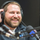Software Development Kit (SDK) and API Discussions

## How to fetch CPU Busy percentage using perf-object-get-instances2,798 Views

I am using perf-object-get-instances to fetch CPU busy percentage with object : system, instance : system and counter : cpu_busy. However, the returned value is 11 digit number.
How do I convert this to percentage ?

Is there any other way to fetch this information ?

1 ACCEPTED SOLUTION2,772 Views

Hello @rohancmr,

In order to get the value you want you need the counter itself (cpu_busy) and the base counter (which I believe is processor_elapsed_time).  You would then need to read the values at two different points in time and do some math...

```# where t1 = first reading and t2 = second reading...
((t2.cpu_busy - t1.cpu_busy) / (t2.processor_elapsed_time - t1.processor_elapsed_time)) * 100 = % CPU busy during that time span```

The base counter, if one is used, is returned back when you query the perf-object-counter-list-info API.

In general there are several different types of counters you'll find...

• raw: single counter value is used
• delta: change in counter value between two samples is used
• rate: delta divided by the time in seconds between samples is used
• average: delta divided by the delta of a base counter is used
• percent: 100*average is used

More information can be found in the ZAPI docs, under the cluster -> perf category.  I also wrote an introduction, using PowerShell, here.

Hope that helps!

Andrew

2 REPLIES 22,773 Views

Hello @rohancmr,

In order to get the value you want you need the counter itself (cpu_busy) and the base counter (which I believe is processor_elapsed_time).  You would then need to read the values at two different points in time and do some math...

```# where t1 = first reading and t2 = second reading...
((t2.cpu_busy - t1.cpu_busy) / (t2.processor_elapsed_time - t1.processor_elapsed_time)) * 100 = % CPU busy during that time span```

The base counter, if one is used, is returned back when you query the perf-object-counter-list-info API.

In general there are several different types of counters you'll find...

• raw: single counter value is used
• delta: change in counter value between two samples is used
• rate: delta divided by the time in seconds between samples is used
• average: delta divided by the delta of a base counter is used
• percent: 100*average is used

More information can be found in the ZAPI docs, under the cluster -> perf category.  I also wrote an introduction, using PowerShell, here.

Hope that helps!

Andrew